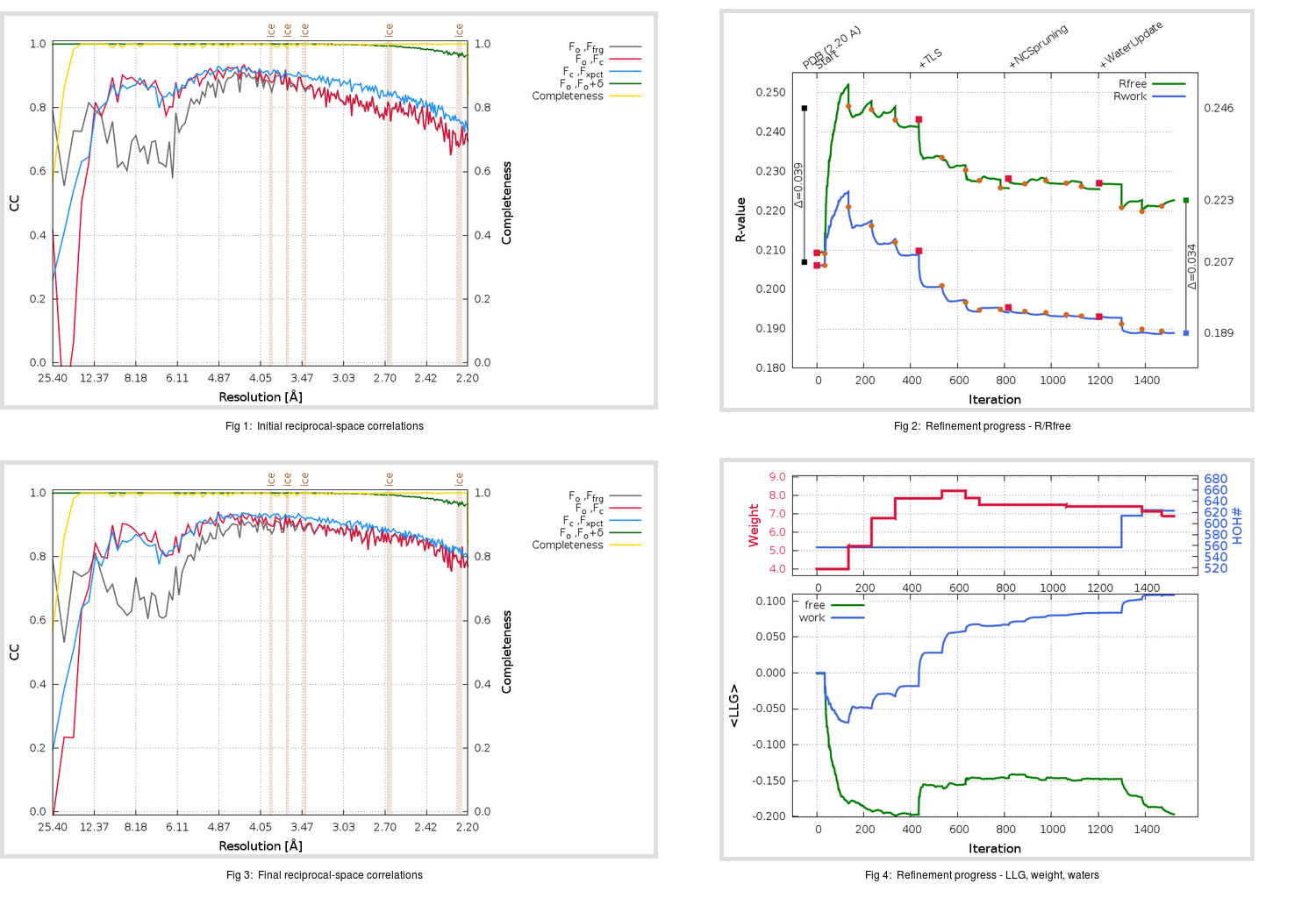Content:

```    Diffraction limits & principal axes of ellipsoid fitted to diffraction cut-off surface:
2.174         1.0000   0.0000   0.0000       0.957 a* - 0.292 c*
2.097         0.0000   1.0000   0.0000       b*
2.169         0.0000   0.0000   1.0000       c*
```

## Deposited

` `
 Date deposited Date data collection Resolution R, Rfree 20090707 20080910 2.20 0.2050 0.2460

Molprobity (CCP4 7.0 version) summary:

```Ramachandran outliers =   0.13 %
favored =  95.37 %
Rotamer outliers      =   3.74 %
C-beta deviations     =     5
Clashscore            =   7.65
RMS(bonds)            =   0.0051
RMS(angles)           =   1.14
MolProbity score      =   2.18
Resolution            =   2.20
R-work                =   0.2050
R-free                =   0.2460
```

```Number of waters      =   557

<B> (all atoms) =   40.86 ( sd =   14.59 ) for       6883 non-hydrogen atoms
<B>   (protein) =   40.67 ( sd =   14.97 ) for       6326 non-hydrogen atoms
<B>     (water) =   43.11 ( sd =    8.97 ) for        557 non-hydrogen atoms
<B>    (others) =    0.00 ( sd =    0.00 ) for          0 non-hydrogen atoms

B min/max       (all non-hydrogen atoms) =   16.29 /   98.21
B min/max   (protein non-hydrogen atoms) =   16.29 /   98.21
B min/max     (water non-hydrogen atoms) =   20.07 /   74.17
B min/max     (other non-hydrogen atoms) =    0.00 /    0.00
```

## BUSTER (re-)refinement

` `

Molprobity (CCP4 7.0 version) summary:

```Ramachandran outliers =   0.26 %
favored =  98.15 %
Rotamer outliers      =   4.04 %
C-beta deviations     =     0
Clashscore            =   3.34
RMS(bonds)            =   0.0113
RMS(angles)           =   1.56
MolProbity score      =   1.59
Resolution            =   2.20
R-work                =   0.1889
R-free                =   0.2227
```

```Number of waters      =   623

<B> (all atoms) =   45.91 ( sd =   17.29 ) for       6949 non-hydrogen atoms
<B>   (protein) =   45.43 ( sd =   17.61 ) for       6326 non-hydrogen atoms
<B>     (water) =   50.78 ( sd =   12.58 ) for        623 non-hydrogen atoms
<B>    (others) =    0.00 ( sd =    0.00 ) for          0 non-hydrogen atoms

B min/max       (all non-hydrogen atoms) =   19.59 /  107.11
B min/max   (protein non-hydrogen atoms) =   19.59 /  107.11
B min/max     (water non-hydrogen atoms) =   23.92 /   95.35
B min/max     (other non-hydrogen atoms) =    0.00 /    0.00
```

Refinement progression:Results:

` `
 File Remark 3I6G_aB_refine.01_04_refine.pdb.gz exact refinement commands are in header 3I6G_aB_refine.01_04_refine.mtz.gz including original deposited data and several re-refinement map coefficients 3I6G_aB_refine.01_04_BUSTER_model.cif.gz including any non-standard compound restraints 3I6G_aB_refine.01_04_BUSTER_refln.cif.gz